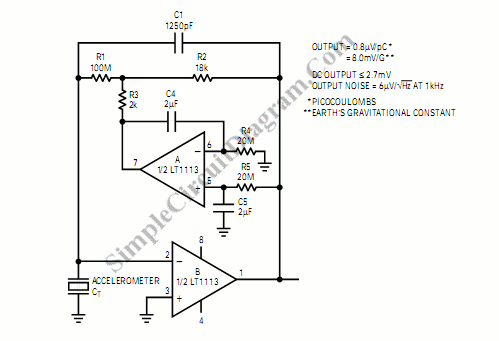# Accelerometer Signal Amplifier

Inverting mode amplifier is needed by precision accelerometers because that usually charge output device. This amplifier is used to convert charge into voltage output. Here is an example of accelerometer with DC servo.C1 is used to convert the charge from the transducer into voltage, which should equal the input capacitance of the op amp plus the transducer capacitance. The value of R1 • C1 (or R1 (1 + R2/R3) for a Tee network) will affect on the low frequency bandwidth of the amplifier. The noise gain will be 1 + C1/CT. Time constant of the amplifier (1/R1C1) must be smaller than the time constant of the servo (1/R5C5). [Schematic diagram source: Linear Technology Application Notes]